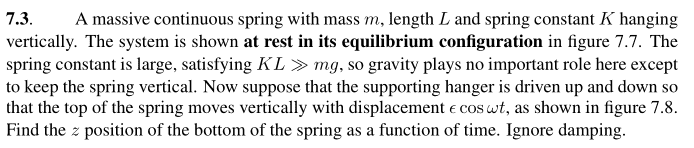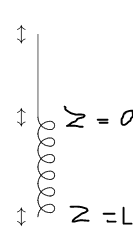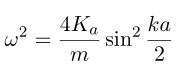# Hanging Spring with non-negligible mass is subjected to a driving force

2020 Award
Homework Statement:
Print below
Relevant Equations:
I will post below.First i will use a equation resulted in considering a spring as a continuum limit of massive mass:

ω = √(KL/ρ)*kn

ρ is the linear density ρ

ω = √(KL/ρ)*kn

X = A*cos(kn*x)*cos(ω*t)

ξ = A (first consequence)

X = ξ*cos(kn*x)*cos(ω*t)

∂y/∂x need to be zero in x=L (for strain be zero)

kn*L = n*π (second)

ω = √(KL/ρ)*kn = √(KL/ρ)*n*π/L

So

X = L + ξ*cos(((n*π))*cos(ω*t)

where

ω = √(K/M)*n*π

n is a natural

This seems okay?

Last edited by a moderator:

## Answers and Replies

haruspex
Science Advisor
Homework Helper
Gold Member
2020 Award
Homework Statement:: Print below
Relevant Equations:: I will post below.

First i will use a equation resulted in considering a spring as a continuum limit of massive mass:
You've lost me already. I don't know what you mean by that, nor how you get that equation, nor what kn is. For the equation to be dimensionally consistent, kn would have to have dimension L-1, right?

I would expect to see a differential equation obtained by considering an element length dy subject to different tensions above and below.

2020 Award
You've lost me already. I don't know what you mean by that, nor how you get that equation, nor what kn is.
I would expect to see a differential equation obtained by considering an element length dy subject to different tensions above and below.
I am trying to approach the problem by means of waves, so when the up part is oscillating, the wave will propagate by the spring until the end of the spring...
I think i need to explain better, kn has the same sense as we use it in string oscillating vertically, it is like the wave number.
And the expression for w is just a result by oscillate the massive spring:

Imagine a spring as a lot of masses in line and as perturbation in one end is made.

You know the result ω will beBut as we are imagining a massive spring,

m is the little mass
Ka is the spring stiffness in the little mass region.

a -> 0

When we cut a spring, the stiffnes of each part is 2K right? Following this thinking:

Ka*a = K*L

ω² = (4*K*L/m*a)*k²*a²/4

ω = √(K*L/ρ)*k
ρ = M/L

and just put in the stationary wave with respectives conditions...

Since the waves propagates in the spring direction, when it came below, it will governs the motion of the end

haruspex
Science Advisor
Homework Helper
Gold Member
2020 Award
Ok, I understand a little better, but it doesn’t seem to me you have arrived at an answer to the question. I would have thought we are supposed to find the phase and amplitude at the free end.
Also, I am confused by your use of x. In one place it looks like x is the distance of an element from one end in the relaxed state, and y is its displacement from its equilibrium position; in another, x seems to be the position of an element as a function of time.
Please define all your variables clearly.

2020 Award
Ok, I understand a little better, but it doesn’t seem to me you have arrived at an answer to the question. I would have thought we are supposed to find the phase and amplitude at the free end.
Also, I am confused by your use of x. In one place it looks like x is the distance of an element from one end in the relaxed state, and y is its displacement from its equilibrium position; in another, x seems to be the position of an element as a function of time.
Please define all your variables clearly.
I agree there is a flaw in approach the problem by this way, because i can not find any k, and i dont know how could we find it.

I will rewrite:

Now y denote the initial position and X the position at time t

ω = √(KL/ρ)*kn
ρ is the linear density ρ

X = y + A*cos(kn*y)*cos(ω*t)

(ξ*cos(ω*t) at y=0)=>

X = ξ*cos(kn*y)*cos(ω*t)

∂X/∂y need to be zero in y=L (for strain be zero)

kn*L = n*π (second)

ω = √(KL/ρ)*kn = √(KL/ρ)*n*π/L

So

X = L + ξ*cos(((n*π))*cos(ω*t)

where
ω = √(K/M)*n*π

Chestermiller
Mentor
If the spring were deformed uniformly, the tension would be $$T=Ku_L$$where ##u_L## is the displacement at x = L, assuming that x = 0 is fixed. This equation can also be written as $$T=KL\frac{u_L}{L}$$where the local uniform strain in the spring is given by $$\epsilon(x)=\frac{u_L}{L}=\frac{\partial u}{\partial x}$$Therefore, in any arbitration non-uniform axial deformation of the spring, the local tension is given by:
$$T(x)=KL\frac{\partial u}{\partial x}$$

For the problem at hand, if we perform a force balance on the portion of the spring between x and ##x+\Delta x##, we obtain:$$T(x+\Delta x)-T(x)=\frac{m}{L}\Delta x\frac{\partial^2u}{\partial t^2}$$Combining the previous two equations, dividing by ##\Delta x##, and taking the limit as ##\Delta x## approaches zero yields:
$$\frac{\partial^2u}{\partial t^2}=\frac{KL^2}{m}\frac{\partial^2u}{\partial x^2}=c^2\frac{\partial^2u}{\partial x^2}$$where c is the wave speed: $$c=L\sqrt{\frac{K}{m}}$$The boundary condition at x = L is zero tension: ##\frac{\partial u}{\partial x}=0## @ x = L.

The solution to this problem is going to be of the form $$u(x,t)=A(x)\cos{\omega t}+B(x)\sin{\omega t}$$subject to the initial conditions ##A(0)=\epsilon##, B(0)=0.

•etotheipi
etotheipi
@Chestermiller just to clarify, have you defined ##x## to be the material coordinates and ##u(x,t)## as the displacement of the point of the spring at material coordinate ##x## from its position at ##t=0##? That is to say that the function which converts the material coordinate to the 'real' coordinate ##X## is ##X(x,t) = x + u(x,t)##?

haruspex
Science Advisor
Homework Helper
Gold Member
2020 Award
The solution to this problem is going to be of the form $$u(x,t)=A(x)\cos{\omega t}+B(x)\sin{\omega t}$$
I worried that there might be harmonics. Is that not possible ?

2020 Award
If the spring were deformed uniformly, the tension would be $$T=Ku_L$$where ##u_L## is the displacement at x = L, assuming that x = 0 is fixed. This equation can also be written as $$T=KL\frac{u_L}{L}$$where the local uniform strain in the spring is given by $$\epsilon(x)=\frac{u_L}{L}=\frac{\partial u}{\partial x}$$Therefore, in any arbitration non-uniform axial deformation of the spring, the local tension is given by:
$$T(x)=KL\frac{\partial u}{\partial x}$$

For the problem at hand, if we perform a force balance on the portion of the spring between x and ##x+\Delta x##, we obtain:$$T(x+\Delta x)-T(x)=\frac{m}{L}\Delta x\frac{\partial^2u}{\partial t^2}$$Combining the previous two equations, dividing by ##\Delta x##, and taking the limit as ##\Delta x## approaches zero yields:
$$\frac{\partial^2u}{\partial t^2}=\frac{KL^2}{m}\frac{\partial^2u}{\partial x^2}=c^2\frac{\partial^2u}{\partial x^2}$$where c is the wave speed: $$c=L\sqrt{\frac{K}{m}}$$The boundary condition at x = L is zero tension: ##\frac{\partial u}{\partial x}=0## @ x = L.

The solution to this problem is going to be of the form $$u(x,t)=A(x)\cos{\omega t}+B(x)\sin{\omega t}$$subject to the initial conditions ##A(0)=\epsilon##, B(0)=0.

I almost get it, unless the X = L + ξ*cos(((n*π))*cos(ω*t) in the equation, so in my way n would be some even number? Why should it be?

2020 Award
This remember the fact when we driven something below resonance frequency, the system resonates with the force, while above the resonance frequency, it will oscillates180 degree out.

Chestermiller
Mentor
@Chestermiller just to clarify, have you defined ##x## to be the material coordinates and ##u(x,t)## as the displacement of the point of the spring at material coordinate ##x## from its position at ##t=0##? That is to say that the function which converts the material coordinate to the 'real' coordinate ##X## is ##X(x,t) = x + u(x,t)##?
Yes, material point, but not at time zero. It is supposed to be distance along the spring at a time when the spring is not under any load. In the present system, the motion is supposed to be a stationary oscillatory motion, so that at each material point, it is periodic, but with a different phase angle.

•etotheipi
Chestermiller
Mentor
I worried that there might be harmonics. Is that not possible ?
I don't think that higher harmonics can satisfy the boundary conditions.

Chestermiller
Mentor
I almost get it, unless the X = L + ξ*cos(((n*π))*cos(ω*t) in the equation, so in my way n would be some even number? Why should it be?
I don't get this result. I get the following standing wave:
$$u(x,t)=\epsilon\frac{\cos{\frac{\omega}{c}(L-x)}}{\cos{\frac{\omega}{c}L}}\cos{\omega t}$$I believe that this satisfies the boundary conditions and the differential equation.

•TSny